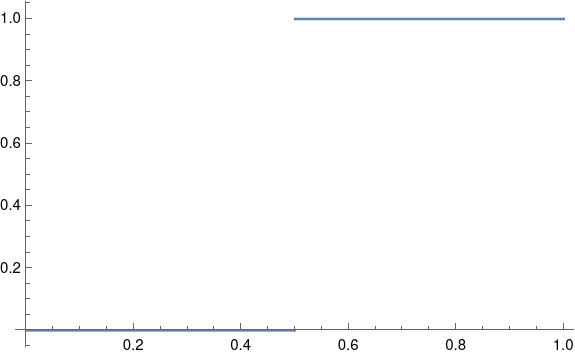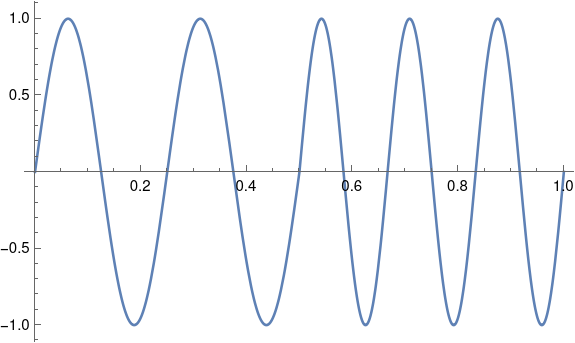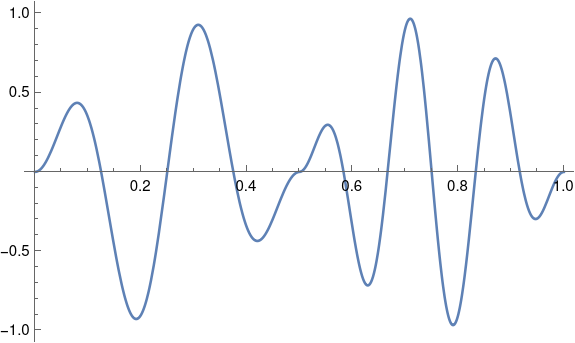# Frequency shift keying (FSK) spectrum

This post will look encoding digital data as an analog signal using frequency shift keying (FSK), first directly and then with windowing. We’ll look at the spectrum of the encoded signal and show that basic FSK uses much less bandwidth than direct encoding, but more bandwidth than FSK with windowing.

## Square waves

The most natural way to encode binary data as an analog signal would be represent 0s and 1s by a sequence of pulses that take on the values 0 and 1.A problem with this approach is that it would require a lot of bandwidth.

In theory a square wave has infinite bandwidth: its Fourier series has an infinite number of non-zero coefficients. In practice, the bandwidth of a signal is determined by how many Fourier coefficients it has above some threshold. The threshold would depend on context, but let’s say we ignore Fourier components with amplitude smaller than 0.001.

As I wrote about here, the nth Fourier sine series coefficients for a square wave is equal to 4/nπ for odd n. This means we would need on the order of 1,000 terms before the coefficients drop below our threshold.

## Frequency shift keying

The rate of convergence of the Fourier series for a function f depends on the smoothness of f. Discontinuities, like the jump in a square wave, correspond to slow convergence, i.e. high bandwidth. We can save bandwidth by encoding our data with smoother functions.

So instead of jumping from 0 to 1, we’ll encode a 0 as a signal of one frequency and a 1 as a signal with another frequency. By changing the frequency after some whole number of periods, the resulting function will be continuous, and so will have smaller bandwidth.

Suppose we have a one second signal f(t) that is made of half a second of a 4 Hz signal and half a second of a 6 Hz signal, possibly encoding a 0 followed by a 1.What would the Fourier coefficients look like? If we just had a 4 Hz sine wave, the Fourier series would have only one component: the signal itself at 4 Hz. If we just had a 6 Hz sine wave, the only Fourier component would again be the signal itself. There would be no sine components at other frequencies, and no cosine components.

But our signal patched together by concatenating 4 Hz and 6 Hz signals has non-zero cosine terms for every odd n, and these coefficients decay like O(1/n²).

Our Fourier series is

f(t) = 0.25 sin 8πt + 0.25 sin 12πt + 0.0303 cos 2πt + 0.1112 cos 6πt – 0.3151 cos 12πt + 0.1083 cos 10πt + …

We need to go out to 141 Hz before the coefficients drop below 0.001. That’s a lot of coefficients, but it’s an order of magnitude fewer coefficients than we’d need for a square wave.

## Pulse shaping

Although our function f is continuous, it is not differentiable. The left-hand derivative at 1/2 is 8π and the right-hand derivative is 12π. If we could replace f with a related function that is differentiable at 1/2, presumably the signal would require less bandwidth.

We could do this by multiplying both halves of our signal by a windowing function. This is called pulse shaping because instead of a simple sine wave, we change the shape of the wave, tapering it at the ends.

Let’s using a cosine window because that’ll be easy; in practice you’d probably use a different window .Now our function is differentiable at 1/2, and its Fourier series converges more quickly. Now we can disregard components above 40 Hz. With a smoother windowing function the windowed function would have more derivatives and we could disregard more of the high frequencies.

## Related posts

 This kind of window is called a cosine window because you multiply your signal by one lobe of a cosine function, with the peak in the middle of the signal. Since we’re doing this over [0. 1/2] and again over [1/2, 1], we’re actually multiplying by |sin 2πt|.

## One thought on “Frequency shift keying (FSK) spectrum”

1. Hi,
Love your series of short posts on FM and related things. Just trying to understand a bit more about how this works and found them a great resource.